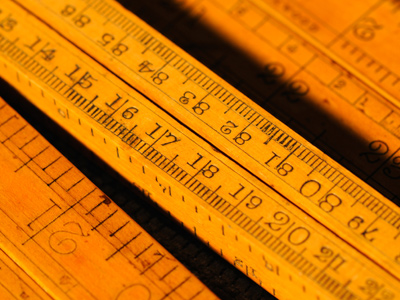There are 10 millimetres in a centimetre.

# Level 5-6 Numbers - Decimals - Multiplication and Division

After addition and subtraction, multiplication and division are the most common parts of maths. That's true in KS3 Maths just as much as it is in junior school or university. Once you've got the hang of multiplication and division it becomes quite easy - even when working with decimals.

Multiplication and division of decimals is very similar to normal multiplying and dividing of numbers. The one thing you need to be aware of is the placement of the decimal point. It might help you to make a guess as to the answer before you do your calculations. For example, if you multiply 2.3 x 4.01 you know that the answer will be something like 9, not 92,000! Better still, learn the rules for placing decimal points in multiplication and division - that will make the problems much easier to solve.

In the following quiz I recommend that you estimate your answers before you do your calculations - that should help! Good luck.

1.
What is 6.04 ÷ 4?
1
1.4
1.5
1.51
6 ÷ 4 =1.5 and 0.4 ÷ 4 = 0.01. Now just add these two figures together
2.
Joanne is building a bookcase and needs 38 fixings at 14p each. How much in total will she pay for the fixings?
£3.40
£5.32
£53.20
£532.00
Remember that 14p = 0.14 when written in decimals!
3.
What is 48.44 x 7?
339.08
340.44
484.47
500
An approximation would be 50 x 7 = 350 so you know straightaway that last two answers are wrong
4.
Joseph spends a total of £34.44 on four new rose bushes for his garden. How much is one rose bush?
£3.40
£3.44
£8.44
£8.61
34 ÷ 4 = 8.5 and 0.44 ÷ 4 = 0.11. Add these two figures together for the answer
5.
There are 10 millimetres in a centimetre. How would you write 34.12cm in millimetres?
3.412mm
34.12mm
341.2mm
3,412mm
Remember - all the digits move one place to the left when multiplying by 10
6.
A t-shirt costs £5.99. Mrs. Crawford bought one each for her four children. How much did she spend?
£20.00
£22.96
£23.96
£24.50
Remember to estimate the answer. £6.00 x 4 = £24.00, so the figure should be close to this
7.
How would you write 655.35mm in centimetres?
65.535cm
655.35cm
6,553.5cm
6,600cm
To divide by 10 just move all the digits one space to the right
8.
What is 1,270.5 ÷ 15?
82.7
84.7
86.7
88.7
You could work this one out in your head by first dividing 1,270.5 by 3 to get 423.5. Next you would divide 423.5 by 10 to get 42.35 (one 30th of 1,270.5). Multiply this number by 2 for the answer: 84.7. Or you could use pen and paper - much easier!
9.
If a tube of toothpaste costs £1.57, how much do three tubes cost?
£3.15
£4.50
£4.66
£4.71
To double-check your answer, £1.57 + £1.57 + £1.57 is the same as £1.57 x 3!
10.
What is 2.42 x 5?
10.2
11.7
12.1
13.5
To multiply by 5 simply move all the digits one place to the left and then halve the answer